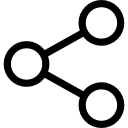Tags:
Hindi# Memory Based Questions IBPS PO Prelims 7th October 2017

## Memory Based Questions IBPS PO Prelims 7th October 2017 PDF Download

All candidates are advised to go through this post and practice those IBPS PO Prelims Memory based questions 7th October 2017 which will help them understand the pattern asked properly.

Let us now go through questions topic- by – topic and try to solve each one of them-

##### Topic- Number Series

1). 17, 98, 26,89, 35, ?

Logic:

• 17+9 = 26; 26+9 = 35
• 98 – 9 = 89;   89-9=80

2). 2, 17, 89, 359, 1079,?

Logic:

• 2*6 + 5 = 17
• 17*5 + 4 = 89
• 89 * 4 + 3 = 359
• 359 * 3 + 2 = 1079
• 1079 * 2 + 1 = 2159

3). 3, 5, 15, 45, 113, ?

Logic:

+1^3+1, +2^3+2, +3^3+3, +4^3+4, +5^3+5

4). 7, 4.5, 5.5, 12, 49, ?

Logic:

• 7 × 0.5 + 1 = 4.5
• 4.5 × 1 + 1 = 5.5
• 5.5 × 2 + 1 = 12
• 12 × 4 + 1 = 49
• 49 × 8 + 1 = 393

5). 3240, 540, 108, 27, ?, 4.5

Logic:

• 3240/6 = 540
• 540/5 = 108
• 108/4 = 27
• 27/3 = 9
• 9/2 = 4.5

IBPS PO Exam Analysis for Prelims and Mains 2017 – Find the question paper review for all shifts and days here.

##### Topic- Puzzle

Seven person A, B, C, D, E, F G likes seven colors. Yellow White Red Orange Blue Gray Black. They visit in different days starting from Monday to Sunday

• A visits one day after Thursday.
• Only 4 people are in between A and B
• The one who likes Red color visits immediately after B.
• Only one person visit between Red and Blue.
• The one who like white color visit before one of days on which day C visits.
• The one who like white color does not visit on Monday.
• Only 1 person is in between D and E. D like Yellow.
• There are same as many person in between A and Blue color which are one less in between B and C.
• Neither G nor F likes Black.
• G does not visit on Saturday and not like gray color .
 Monday D Yellow Tuesday B White Wednesday E Red Thursday G Orange Friday C Blue Saturday F Grey Sunday A Black

Puzzle Set-2:

J, K, L, M, N, O and P are seven different boxes of different colors i.e. Brown, Orange, Silver, Pink, Yellow, White and Green but not necessarily in the same order.
Box which is of Brown color is immediately above J. There are only two box between M and the box which is of Brown color. Box which is of Silver color is above M but not immediately above M. Only three box are between L and the box which is of Silver color.
The box which is of Green color is immediately above L. The box which is of Pink color is immediately above the box P. Only one box is there between K and N. Box K is above N. Neither box K nor J is of Yellow color. J is not of orange color.

• O- Silver
• K- Orange
• M- Yellow
• N- Green
• L- Pink
• P- Brown
• J- White
##### Topic- Syllogism

Direction (1-2):

Statement:

• All remarks are feedbacks
• Some feedbacks are words
• No word is a digit

1). Conclusion:

• Some Feedbacks are definitely not digits.
• All digits being feedbacks is a possibility.

2). Conclusion:

• All remarks being words is a possibility
• At least some remarks are digits.

3). Statements:

• Some files are boxes
• All boxes are carton
• No carton is a plastic

Conclusion:

• No file is a plastic.
• Some files are plastics.

4). Statement:

• Some desks are chairs
• Some chairs are seats
• No seat is a table

Conclusion:

• All desks can never be table
• Some chains are definitely not tables.

5). Statements:

• All routes are ways
• All ways are paths
• Some ways are bridges

Conclusion:

• At least some bridges are routes
• All routes being bridge is a possibility.
##### Topic- Approximation

1.(√80.997 – √25.001) x (√120.98 + √16.02) = ?

• ? = (√81 – √25) x (√121 + √16)
• ? = (9 – 5) x (11 + 4)
• ? = 4 x 15 = 60
• ? = 60

2.55.01 – 345.02÷ 22.99 × 2 = ?

• ? = 55 – 345 ÷ 23 × 2 = 55 – 30
• ? = 15

3.(184.002 – 29 ÷ 5)x 29.997 = X

• (184 – (29/5)) x 30 = X
• X = 5340

4.√(3099.985÷62.001+14.001) = X

• √3100/62 + 14 = X
• √50+ 14 = X
• √64 =X
• X = 8

5.(111.91 x51) ÷14.02 = 11.002 + X

• (112 x 51)/14– 11 = X
• X = 397

6.(84.01 of 24. 97% ÷ 6.995) = ?

• ? = 84 x 1/4 ÷ 7
• ?= 21/7 = 3
• ? = 3
abhishek tripathi 1 Months ago
Thanks
manish panjwani 1 Months ago
some solutions are wrong